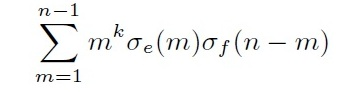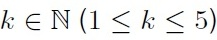##### Hermite-Hadamard and Simpson Type Inequalities for Differentiable Harmonically P-functions

Imdat Işcan, Selim Numan, Kerim Bekar

Journal of Advances in Mathematics and Computer Science, Page 1908-1920
DOI: 10.9734/BJMCS/2014/10338

In this paper, we introduce the concept of the harmonically P-functions, give Hermite-Hadamard’s inequalities for harmonically P-functions and define a new identity. By using this identity, we obtain new estimates on generalization of Hadamard and Simpson type inequalities for harmonically P-functions.

##### Implementing a Matrix Vector Transition Net

Tony Spiteri Staines

Journal of Advances in Mathematics and Computer Science, Page 1921-1940
DOI: 10.9734/BJMCS/2014/10349

Aims: Classic Petri nets also known as place transition nets provide many interesting and useful features for system modeling. However they are limited by the place types that are used. An approach is presented where a matrix vector transition net is used for modeling complex system behavior. This is more expressive than a Petri net.
Proposed Solution: A modified form of a traditional place transition net is used. The idea is to combine the ideas from Petri net behavior with the matrix vector approach.
Implementing the Matrix Vector Transition Net: Ordinary Petri net places are replaced with matrices or vectors. The input and output arcs have a specific function matrix that determines firing. Firing and behavior remains conceptually similar to that of a petri net. Row and column vectors can also be used interchangeably. The behavior of matrix transition nets is similar to that of a place transition net. Instead of tokens, matrix elements are used. This type of structure increases the modeling power, abstraction capacity and the complexity of the net.
Case Study: A toy case of an abstract network structure containing processing elements is used to illustrate the use of the matrix vector transition net structure.
Results and Findings: The behavior of matrix transition nets is shown to be similar to that of a place transition net. Instead of tokens, matrix elements are used. It is possible to construct a symbolic marking graph or reachability graph for the system This type of structure definitely increases the modeling power, abstraction capacity and the complexity of the net. The matrix transition net could be useful for certain types of communication system problems and complex system interfacing.

##### Two-step Iterative Methods with Sixth-order Convergence for Solving Nonlinear Equations

R. Thukral

Journal of Advances in Mathematics and Computer Science, Page 1941-1950
DOI: 10.9734/BJMCS/2014/10214

Two parameter families of sixth-order iterative methods for finding simple zeros of nonlinear equations are developed. The new methods have the order of convergence order of five or six. Per iteration these new methods require two evaluations of the function and two evaluations of the first-order derivatives. In fact, the efficiency index of these methods is. Several examples are given to illustrate the efficiency of these new methods and their comparisons with other sixth-order method.

##### Intuitionistic Trapezoidal Fuzzy Prioritized Operators and Their Application to Multiple Attribute Group Decision Making

Zhiming Zhang

Journal of Advances in Mathematics and Computer Science, Page 1951-1998
DOI: 10.9734/BJMCS/2014/10133

Aims: The aim of this paper is to propose some intuitionistic trapezoidal fuzzy prioritized operators and interval-valued intuitionistic trapezoidal fuzzy prioritized operators for handling multiple attribute group decision making problems in which the attributes and decision makers are in different priority level.
Study Design: First, we present two intuitionistic trapezoidal fuzzy prioritized aggregation operators called the intuitionistic trapezoidal fuzzy prioritized weighted average (ITFPWA) operator and the intuitionistic trapezoidal fuzzy prioritized weighted geometric (ITFPWG) operator.
Place and Duration of Study: These two operators can capture the prioritization phenomenon among the aggregated arguments.
Methodology: Then, some desirable properties of two new operators are examined in detail. Next, we utilize the proposed operators to develop an approach to multiple attribute group decision making under intuitionistic trapezoidal fuzzy environment in which the attributes and decision makers are in different priority level, and a practical example is provided to illustrate the practicality and effectiveness of the developed approach.
Results: Furthermore, the intuitionistic trapezoidal fuzzy prioritized aggregation operators are extended to interval-valued intuitionistic trapezoidal fuzzy environment, and two interval-valued intuitionistic trapezoidal fuzzy prioritized aggregation operators are introduced. Subsequently, an approach to multiple attribute group decision making with interval-valued intuitionistic trapezoidal fuzzy information is developed.
Conclusion: Finally, an application of the proposed approach to group decision making problem with interval-valued intuitionistic trapezoidal fuzzy information is shown with the demonstration of a numerical example.

##### On the Properties of the Set Solutions of a Class of Paratingent Equation with Delay

Lotfi Boudjenah

Journal of Advances in Mathematics and Computer Science, Page 1999-2003
DOI: 10.9734/BJMCS/2014/10537

In this paper we will study the main properties of the set solutions of the paratingent equation (type differential inclusion) with delay of the form: (Ptx)(t) F([x]t) for t ≥ 0 with the initial condition: x(t) = ξ(t) for t ≤ 0. We will be interested particularly in the topological properties of the set solutions and the continuous dependence on initial conditions of the set solutions.

##### The Convolution Sums ∑n−1 m=1 mk σe (m) σf(n-m)

Aeran Kim

Journal of Advances in Mathematics and Computer Science, Page 2004-2035
DOI: 10.9734/BJMCS/2014/9704

In this paper we obtain some various convolution sum formulae especially, focusing on the formforand an odd positive integer e and f. Furthermore, we obtain some identities related to the convolution sums.

##### Vaccination Dynamics of Chickenpox in Agona West Municipality of Ghana

Ahmed Baffoe Esson, William Obeng-Denteh, Benedict Barnes, Godfrey E. Ntherful

Journal of Advances in Mathematics and Computer Science, Page 2036-2045
DOI: 10.9734/BJMCS/2014/7883

The study showed that chickenpox cases in recent times have increased in Agona West Municipality with R0=2. We estimated the reproductive number of chickenpox with vaccination to be R0=0.3<1. This showed that after vaccination, the disease will die out. It was estimated that 46.2% corresponding to 53,241 of the people needs to be vaccinated in order to control the chickenpox. The stability analysis of disease free and endemic equilibrium point of chickenpox transmission with vaccination was estimated to be unstable and asymptotically stable. Sensitivity analysis of the SEIR model with vaccination showed that when vaccination rate coefficient is increased chickenpox will be under control.

##### Attributes Reduction Based on Interval-Valued Fuzzy Rough Sets

Zhiming Zhang

Journal of Advances in Mathematics and Computer Science, Page 2046-2066
DOI: 10.9734/BJMCS/2014/10426

Aims: This paper provides a systematic study on attribute reduction with interval-valued fuzzy rough sets.
Study Design: The interval-valued fuzzy rough sets are an important improvement of traditional rough set model to deal with both fuzziness and vagueness in data which the traditional one cannot handle.
Place and Duration of Study: The existing researches on interval-valued fuzzy rough sets mainly focus on the establishment of lower and upper approximation operators by using constructive and axiomatic approaches. Less effort has been put on the attributes reduction of databases based on interval-valued fuzzy rough sets.
Methodology: After introducing some concepts and theorems of attributes reduction with interval-valued fuzzy rough sets, we study the structure of the attributes reduction with interval-valued fuzzy rough sets and present an algorithm by using discernibility matrix to find all the attributes reductions with interval-valued fuzzy rough sets.
Results: Finally, we propose an example to demonstrate our idea and method in this paper.
Conclusion: With these discussions we construct a basic foundation for attributes reduction based on interval-valued fuzzy rough sets.

##### Estimation of Pareto Parameters Using a Fuzzy Least-Squares Method and Other Known Techniques with a Comparison

Hegazy M. Zaher, Ahmed A. El-Sheik, Noura A. T. Abu El-Magd

Journal of Advances in Mathematics and Computer Science, Page 2067-2088
DOI: 10.9734/BJMCS/2014/10890

The purpose of this paper is to obtain the fuzzy least-squares estimator for the two-parameter Pareto distribution and to compare the fuzzy estimator with different types of estimators. The trimmed linear moments (TL-moments), linear moments (L-moments) and linear quantile moments (LQ-moments) formulas will be obtained for the two-parameter Pareto distribution and the TL-moments estimator, L-moments estimator and LQ-moments estimator will be derived for the Pareto distribution. Numerical comparisons between the proposed method and the existing methods are implemented. According to these comparisons, it is suggested that the proposed fuzzy least-squares estimator is preferable all times.

##### Positive Solutions of Singular Dirichlet Boundary Value Problems for Second Order Impulsive Differential Equations

Ying He

Journal of Advances in Mathematics and Computer Science, Page 2089-2096
DOI: 10.9734/BJMCS/2014/10859

In this paper, we study the positive solutions of nonlinear singular two-point boundary value problems for second-order impulsive differential equations. The existence of positive solutions is established by using the fixed point theorem in cones.